## Tetrahedron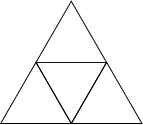The regular tetrahedron, often simply called the'' tetrahedron, is the Platonic Solid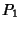with four Vertices, six Edges, and four equivalent Equilateral Triangular faces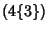. It is also Uniform Polyhedron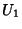. It is described by the Schläfli Symbol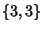and the Wythoff Symbol is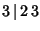. It is the prototype of the Tetrahedral Group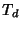,

The tetrahedron is its own Dual Polyhedron. It is the only simple Polyhedron with no Diagonals, and cannot be Stellated. The Vertices of a tetrahedron are given by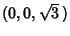,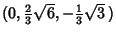,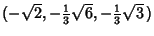, and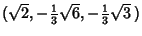, or by (0, 0, 0), (0, 1, 1), (1, 0, 1), (1, 1, 0). In the latter case, the face planes are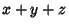(1)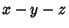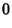(2)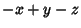(3)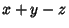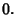(4)

Let a tetrahedron be lengthon a side. The Vertices are located at (, 0, 0), (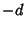,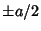, 0), and (0, 0,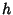). From the figure,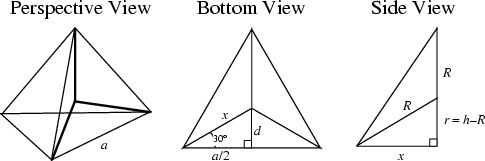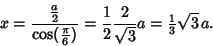(5)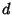is then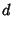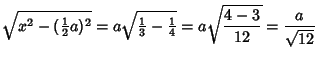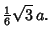(6)

This gives the Area of the base as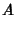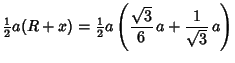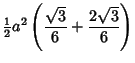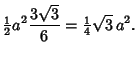(7)

The height is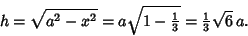(8)

The Circumradius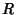is found from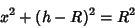(9)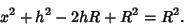(10)

Solving gives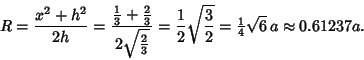(11)

The Inradius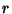is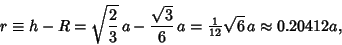(12)

which is also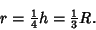(13)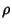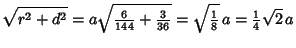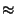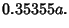(14)

Plugging in for the Vertices gives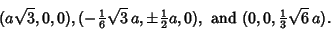(15)

Since a tetrahedron is a Pyramid with a triangular base,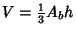, and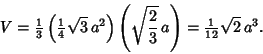(16)

The Dihedral Angle is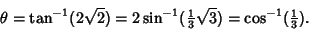(17)

By slicing a tetrahedron as shown above, a Square can be obtained. This cut divides the tetrahedron into two congruent solids rotated by 90°.

Now consider a general (not necessarily regular) tetrahedron, defined as a convex Polyhedron consisting of four (not necessarily identical) Triangular faces. Let the tetrahedron be specified by its Vertices at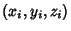where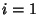, ..., 4. Then the Volume is given by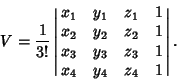(18)

Specifying the tetrahedron by the three Edge vectors a, b, and c from a given Vertex, the Volume is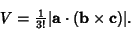(19)

If the faces are congruent and the sides have lengths,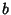, and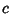, then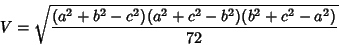(20)

(Klee and Wagon 1991, p. 205). Let,,, andbe the areas of the four faces, and define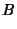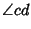(21)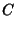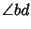(22)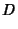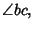(23)

where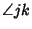means here the Angle between the Planes formed by the Faces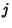and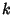, with Vertex along their intersecting Edge. Then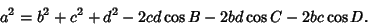(24)

The analog of Gauss's Circle Problem can be asked for tetrahedra: how many Lattice Points lie within a tetrahedron centered at the Origin with a given Inradius (Lehmer 1940, Granville 1991, Xu and Yau 1992, Guy 1994).

See also Augmented Truncated Tetrahedron, Bang's Theorem, Ehrhart Polynomial, Heronian Tetrahedron, Hilbert's 3rd Problem, Isosceles Tetrahedron, Sierpinski Tetrahedron, Stella Octangula, Tetrahedron 5-Compound, Tetrahedron 10-Compound, Truncated Tetrahedron

References

Davie, T. The Tetrahedron.'' http://www.dcs.st-and.ac.uk/~ad/mathrecs/polyhedra/tetrahedron.html.

Granville, A. The Lattice Points of an-Dimensional Tetrahedron.'' Aequationes Math. 41, 234-241, 1991.

Guy, R. K. Gauß's Lattice Point Problem.'' §F1 in Unsolved Problems in Number Theory, 2nd ed. New York: Springer-Verlag, pp. 240-241, 1994.

Klee, V. and Wagon, S. Old and New Unsolved Problems in Plane Geometry and Number Theory, rev. ed. Washington, DC: Math. Assoc. Amer., 1991.

Lehmer, D. H. The Lattice Points of an-Dimensional Tetrahedron.'' Duke Math. J. 7, 341-353, 1940.

Xu, Y. and Yau, S. A Sharp Estimate of the Number of Integral Points in a Tetrahedron.'' J. reine angew. Math. 423, 199-219, 1992.